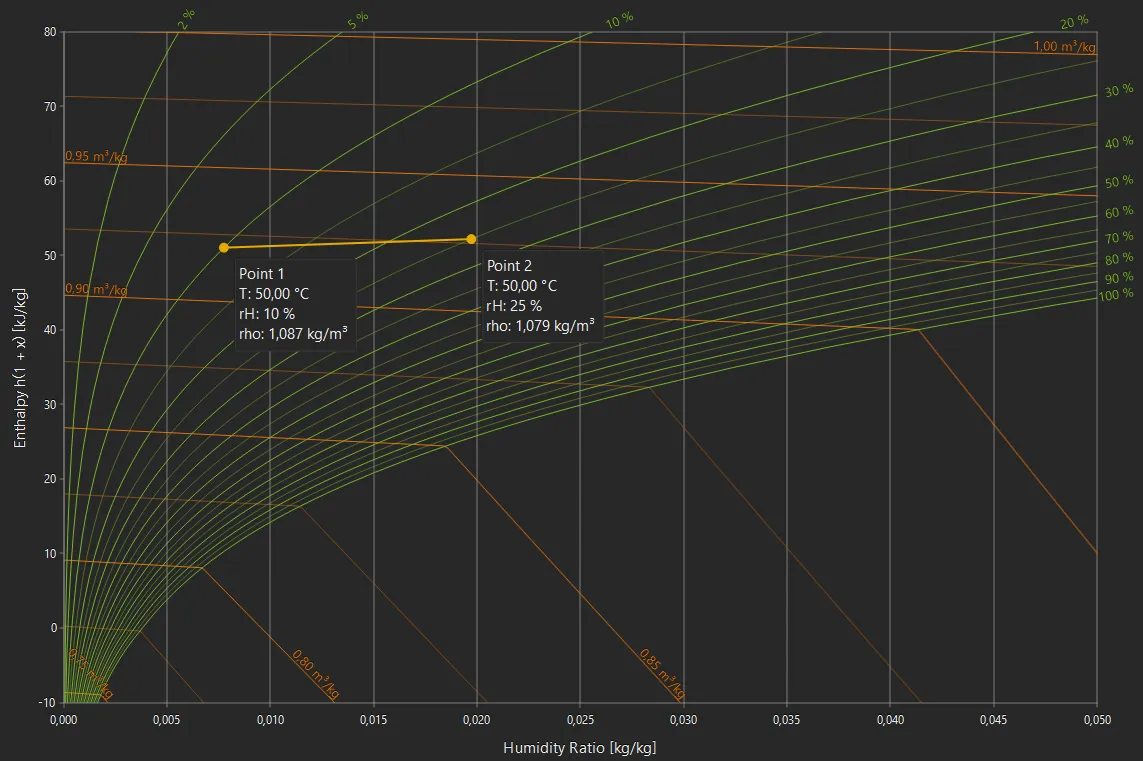# Air Density

bar

°C

%

kg/m³

## How is the air density calculated?

The density of air depends on pressure, temperature and humidity. Dry air at 20°C and standard atmospheric pressure (1.013 bar) has a density of 1.204 kg/m³.

Air behaves like an ideal gas and the density $$\rho$$ (rho) can be calculated accordingly with the following formula:
$$\rho = \frac{p \cdot M}{R \cdot T}$$
Where $$p$$ is the absolute pressure in Pascal (Pa), $$M$$ is the molar mass of air in (kg/mol), $$R$$ is the universal gas constant, and $$T$$ is the temperature in Kelvin (K).

The density of air therefore increases with increasing pressure and decreases with increasing temperature. The lower density of warm air is also the reason why a hot air balloon rises.## Density of humid air

The density of air also depends on the molar mass and thus on the composition of the air. The dry air density at sea level is calculated with a molar mass of about $$M_{\rm A}$$ = 28.96 g/mol.

As water has a lower molar mass with $$M_{\rm W}$$ = 18.02 g/mol, the density of air decreases with increasing humidity.

The molar mass of moist air is calculated from the mass fractions and molar masses of dry air and water:
$$M = x_{\rm A} \, M_{\rm A} + x_{\rm W} \, M_{\rm W}$$
The mass fraction $$x_{\rm W}$$ of water can be calculated from the relative humidity rH and the saturated vapor pressure $$p_{\rm sat}$$:
$$x_W = {\rm rH} \cdot \frac{p_{\rm sat}}{p}$$
The saturated steam pressure $$p_{\rm sat}(T)$$ in turn is a function of temperature. Here we use the Antoine equation for calculation.

For humid air we also have a dew point calculator and a detailed hx diagram.